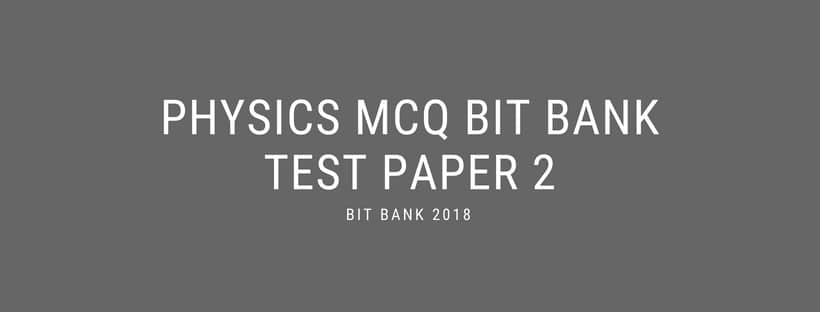Home > Fdaytalk Test > Physics Objective Questions For Competitive Exams- Test Paper 2

# Physics Objective Questions For Competitive Exams- Test Paper 2# Physics Objective Questions For Competitive Exams

## PHYSICS MCQ TEST PAPER 2

Learn Physics Objective Questions For Competitive Exams | Group 1 2 3| Banks | RRB’s

Q) First Person to record sound on steel plates

a) Bell

b) Poulsen

c) Newton

b) Poulsen

Q) Frequency is measured by

a) Speedometer

b) Tonometer

c) Machometer

b) Tonometer

Q) In which one of the following days the speed of sound is more

a) Wet day @ 0 degree Celsius

b) Wet day @ 30 degree Celsius

c) Dry day @ 30 degree Celsius

b) Wet day @ 30 degree Celsius

Q) Intensity of sound is

a) Independent of amplitude

b) Dependent of frequency

c) Independent of frequency

c) Independent of frequency

Q) Units of Intensity

a) Bell

b) Joule

c) Watt

a) Bell

Q) In which one of the material the speed of the sound is more

a) Air

b) Water

c) Plastic Materials

b) Water

Q) The quantity of energy present in the proton is

a) E = hv

b) E = h/v

c) E = he

b) E = h/v

Q) Abbe Georges lemaitre proposed Big Bang theory and its proved by Hubble by using

a) Electric effect

b) Magnetic effect

c) Doppler effect

c) Doppler effect

Q) Speed of the flying can be recorded with

a) Machometer

b) Speedometer

c) Anometer

a) Machometer

Q) DVD refer to

a) Digit Versatile Disc

b) Digital Versatile Disc

c) Digital Verse Disc

a) Digit Versatile Disc

Q) Death of person occurs if intensity of sound exceeds

a) 120 dB

b) 135 dB

c) 125 dB

a) 120 dB

Q) Study of sound is

a) Soundtics

b) Speedometics

c) Acoustics

c) Acoustics

Q) Pitch of sound is _____ of amplitude of sound

a) Equall

b) independent

c) Dependent

b) independent

Q) Salt water is also called as

a) Ocean water

b) Base water

c) Brine solution

c) Brine solution

Q) According Newton, the light properties in the form of minute particles are

a) corpuscles

b) Minute Particles

c) Newtonic particles

a) corpuscles

Q) Normal Sound limit of humans

a) 75 dB

b) 65 dB

c) 60 dB

b) 65 dB

Q) Red shift means

a) Contraction of universe

b) Expansion of universe

c) Unusall Contraction universe

b) Expansion of universe

Q) Study of paper

a) Papeology

b) Paperology

c) Papyrology

c) Papyrology

Q) Study of humidity

a) Hyposcopy

b) Hymnology

c) Hygroscopy

c) Hygroscopy

Q) Quantum theory of radiation was proposed by

a) Max planck

b) Newton

c) Bell

a) Max planck

eBook Code: PHYSICS_MCQ

For More Physics Test Papers – Physics MCQ for exams

Link 3 – 1000+ MCQ Test Papers

Link 5 – Top Books for Competitive Exams

Physics Objective Questions For Competitive Exams

Related Search

Physics mcq gk bits, physics gk bits, physics online bits for exams, physics bits mcq for competitive exams, physics gk gs bits, physics exams mcq gk online, physics pdf mcq questions, physics online gk bits

## 3 thoughts on “Physics Objective Questions For Competitive Exams- Test Paper 2”